• 2021-04-21 16:33:34

plot 是绘制二维图形的最基本函数，它是针对向量或矩阵的列来绘制曲线的。也就是说，使用plot 函数之前，必须首先定义好曲线上每一点的x 及y 坐标。

1. plot(x)

当x 为一向量时，以x 元素的值为纵坐标，x 的序号为横坐标值绘制曲线。当x 为一实矩阵时，则以其序号为横坐标，按列绘制每列元素值相对于其序号的曲线。

2. plot(x，y)

以x 元素为横坐标值，y 元素为纵坐标值绘制曲线

3. plot(x，y1，x，y2，…。)

plot(x，y1，x，y2，…) 以公共的x 元素为横坐标值，以y1，y2，… 元素为纵坐标值绘。

制多条曲线

4. 绘图参数表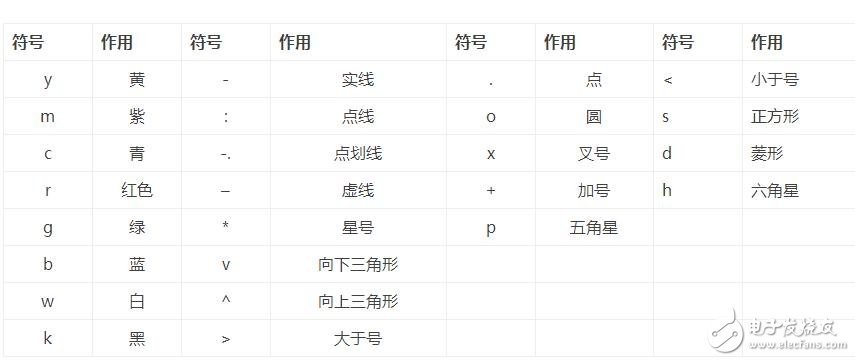例如

plot(x，y1，‘r+-’，x，y2，‘k*：’)1

函数含义

grid on (/off) 给当前图形标记添加(取消)网络

xlable(‘string’) 标记横坐标

ylabel(‘string’) 标记纵坐标

TItle(‘string’) 给图形添加标题

text(x，y，’string’) 在图形的任意位置增加说明性文本信息

gtext(‘string’) 利用鼠标添加说明性文本信息

axis(［xmin xmax ymin ymax］) 设置坐标轴的最小最大值

更多相关内容
• ( 1 ) plot3函数的基本用法 plot3(x，y，z) 其中，参数x、y、z组成一组曲线的坐标。 例1：绘制一条空间折线。 x = [0.2 1.8 2.5]; y = [1.3 2.8 1.1]; z = [0.4 1.2 1.6]; plot3(x,y,z) title('三维曲线','color','r...1、plot3函数
( 1 ) plot3函数的基本用法
plot3(x，y，z)

其中，参数x、y、z组成一组曲线的坐标。

例1：绘制一条空间折线。

x = [0.2 1.8 2.5];
y = [1.3 2.8 1.1];
z = [0.4 1.2 1.6];
plot3(x,y,z)
title('三维曲线','color','r')
grid on
axis([0,3,1,3,0,2])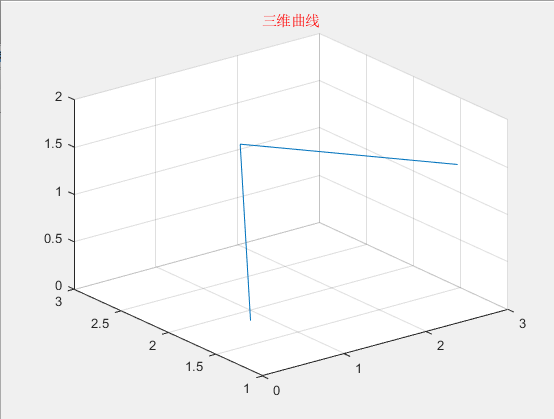t = linspace(0,14*pi,200);
x = sin(t)+t.*cos(t);
y = cos(t)-t.*sin(t);
z = t;

subplot(1,2,1)
plot3(x,y,z)    %有200个数据点，曲线较光滑
grid on

subplot(1,2,2)
plot3(x(1:3:200),y(1:3:200),z(1:3:200))   %有67个数据点，曲线较粗糙
grid on

% subplot(1,2,2)
% t1 = linspace(0,14*pi,80);
% x1 = sin(t1)+t1.*cos(t1);
% y1 = cos(t1)-t1.*sin(t1);
% z1 = t1;
% plot3(x1,y1,z1)
% grid on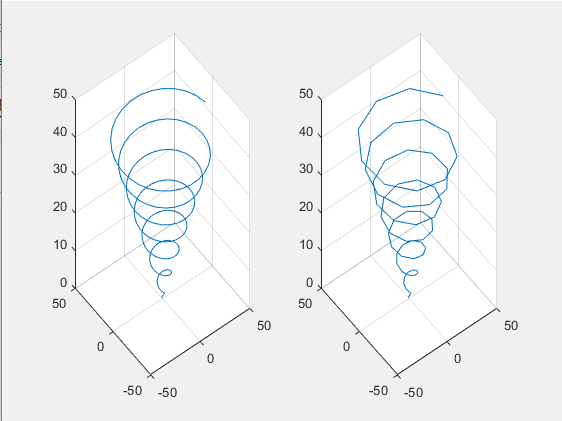( 2 ) plot3函数参数的变化形式
plot3(x, y,z)

①参数x、y、z是同型矩阵：则以XYZ对应列元素绘制曲线，曲线条数等于短阵列数。
②参数x、y、z中有向量，也有矩阵：向量的长度应与矩阵相符，行向量的长度与矩阵的列数相同，列向量的长度与矩阵的行数相同。

例3：在空间不同位置绘制5条正弦曲线。

方法1  列向量
t = linspace(0,2*pi,100);
t = t';
x = [t t t t t];
y = [sin(t) sin(t)+1 sin(t)+2 sin(t)+3 sin(t)+4];
z = [t t t t t];
plot3(x,y,z)
grid on
legend('sin(t)','sin(t)+1','sin(t)+2','sin(t)+3','sin(t)+4','location','north')

方法2  行向量
t = linspace(0,2*pi,100);
x = t;
y = [sin(t);sin(t)+1;sin(t)+2;sin(t)+3;sin(t)+4];
z = t;
plot3(x,y,z)
grid on
legend('sin(t)','sin(t)+1','sin(t)+2','sin(t)+3','sin(t)+4','location','north')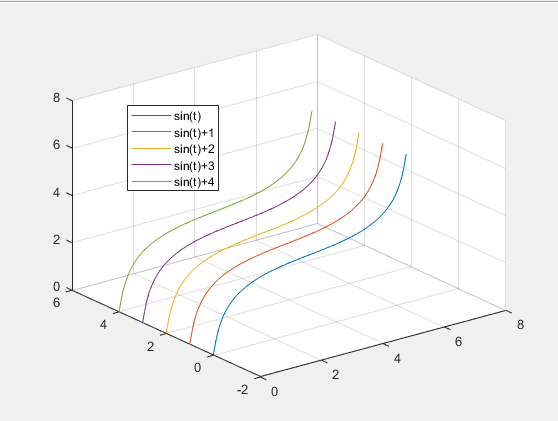(3)含多组输入参数的plot3函数
plot3(x1, y1, z1,x2,y2,z2，…, xn, yn, zn)

每一组x、y、z向量构成一组数据点的坐标，绘制一条曲线。

例4：绘制三条不同长度的正弦曲线。

t1 = 0:0.01:1*pi;
t2 = 0:0.01:2*pi;
t3 = 0:0.01:3*pi;
plot3(t1,sin(t1),t1,t2,sin(t2)+1,t2,t3,sin(t3)+2,t3)
legend('sin(t1)','sin(t2)+1','sin(t3)+2','location','north')
grid on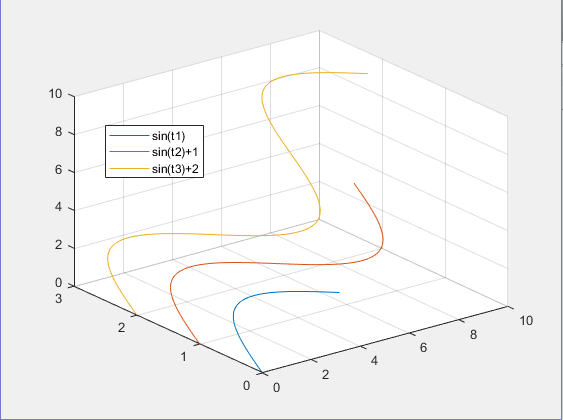(4)含选项的plot3函数
plot3(x, y,z,选项)

选项用于指定曲线的线型、颜色和数据点标记。t = 0:pi/50:6*pi;
x = cos(t);
y = sin(t);
z = 2*t;
plot3(x,y,z,'p')   %p以五角星输出
xlabel('X')
ylabel('Y')
zlabel('Z')
grid on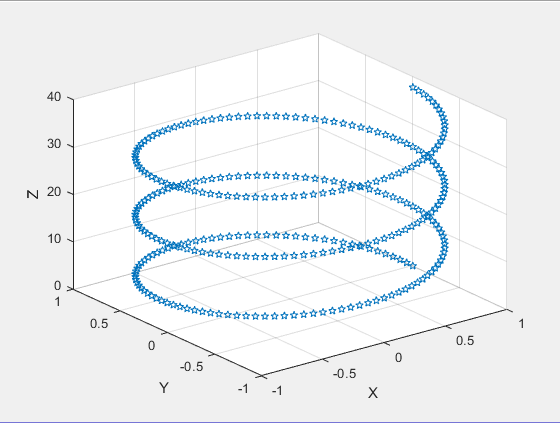2、fplot3函数
fplot3(funx, funy, funz, tlims)
其中, funx、funy、funz代表定义曲线x、y、z坐标的函数，通常采用函数句柄的形式。tlims为参数函数自变量的取值范围，用二元向量[tmin，tmax]描述﹐默认为[-5，5]。xt = @(t)exp(-t/10).*sin(5*t);
yt = @(t)exp(-t/10).*cos(5*t);
zt = @(t)t;
fplot3(xt,yt,zt,[-12,12],'b')
xlabel('X')
ylabel('Y')
zlabel('Z')
title('墨西哥冒顶曲线','fontsize',15,'color','r')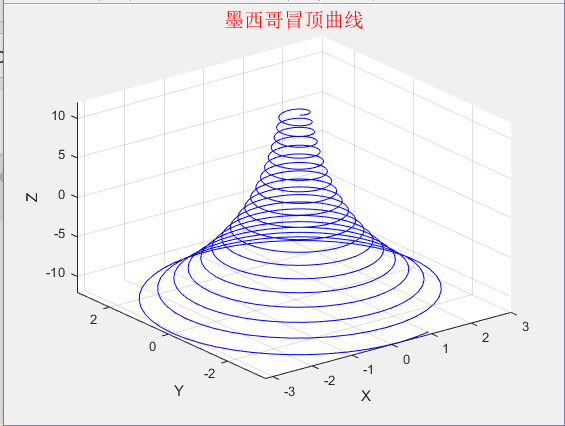展开全文matlab
• plot3函数与plot函数使用方式相近。plot函数具体可参见：https://blog.csdn.net/Mrweng1996/article/details/104338453 1、plot3函数的基本用法： plot3(x, y, z)：其中，x、y、z组成一组曲线的坐标。 代码示例...

plot3函数与plot函数的使用方式相近。plot函数具体可参见：https://blog.csdn.net/Mrweng1996/article/details/104338453

### 1、plot3函数的基本用法：

plot3(x, y, z)：其中，x、y、z组成一组曲线的坐标。

代码示例：

t = linspace(0,10*pi,200);
x = sin(t) + t.*cos(t);
y = cos(t) - t.*sin(t);
z = t;
subplot(1,2,1);
plot3(x, y, z);
grid on
subplot(1, 2, 2);
plot3(x(1:4:200), y(1:4:200), z(1:4:200));
grid on

运行结果：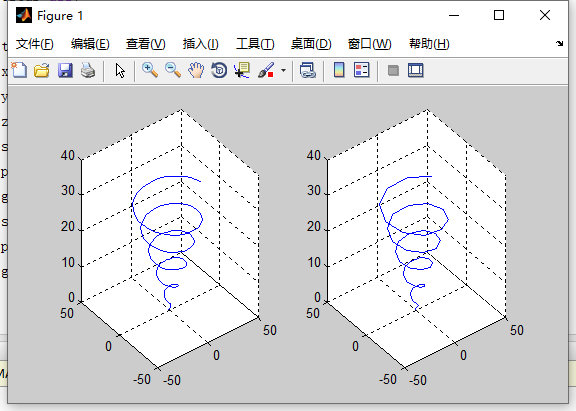### 2、 plot3函数参数的变化形式

plot3(x, y, z)

参数x，y，z为同型矩阵。

参数x，y，z中有向量，有矩阵。

代码示例：

clc;
clear all;

t = 0:0.01:2*pi;
t = t';
x = [t,t,t,t,t];
y = [sin(t),sin(t)+1,sin(t)+2,sin(t)+3,sin(t)+4];
z = [t,t,t,t,t];
plot3(x,y,z);

运行结果：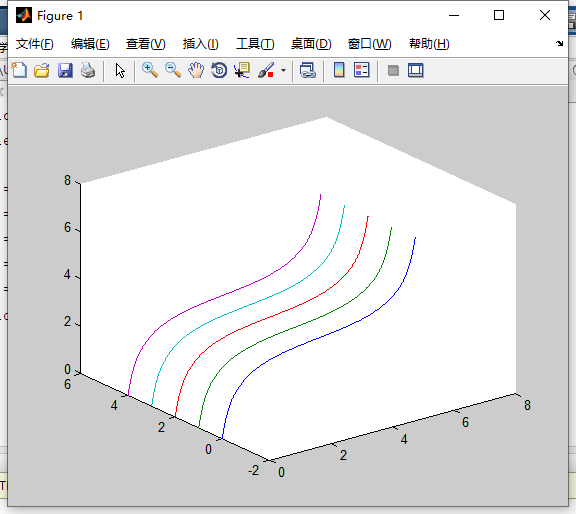### 3、含有多组输入参数的plot3函数

plot3(x1, y1, z1, x2, y2, z2 ..., xn, yn, zn) ：每一组x、y、z组成一组数据点的坐标，绘制一条曲线。

clc;
clear all;

t1 = 0:0.01:1.5*pi;
t2 = 0:0.01:2*pi;
t3 = 0:0.01:3*pi;
plot3(t1, sin(t1), t1, t2, sin(t2)+1, t2,t3, sin(t3)+2, t3)
grid on

运行结果：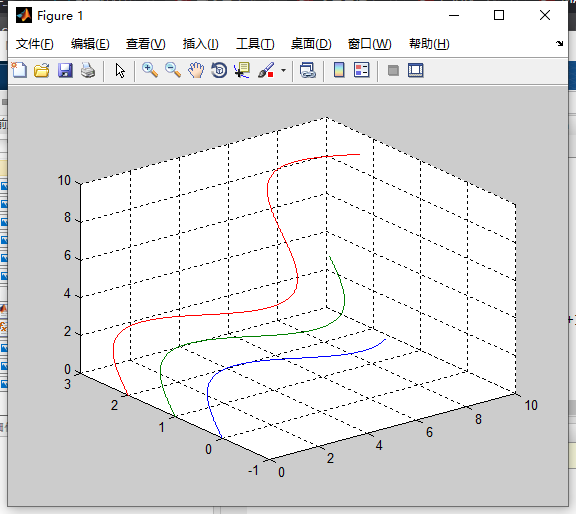### 4、含有选项的plot3函数

plot(x, y, z, 选项)：选项适用于指定曲线的线型、颜色和数据点标记。

代码示例：

clc;
clear all;

t = 0:pi/50:6*pi;
x = cos(t);
y = sin(t);
z = 2*t;
plot3(x, y, z, 'p');
xlabel('X')
ylabel('Y')
zlabel('Z')
grid on


运行结果：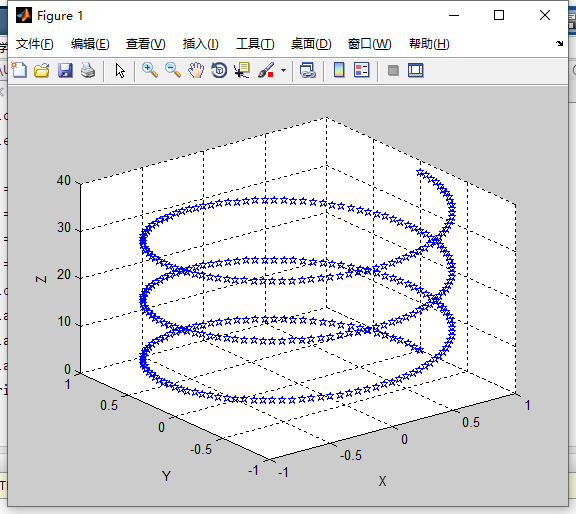展开全文• ## MATLAB中plot函数的用法

万次阅读 多人点赞 2018-04-23 15:47:04
使用plot绘制二维图像本文转自http://blog.sina.com.cn/s/blog_d8f783c90102woqb.html以及...本文仅介绍plot函数的基本用法——使用plot函数绘制二维点图和线...

## 使用plot绘制二维图像

本文转自http://blog.sina.com.cn/s/blog_d8f783c90102woqb.html

以及https://blog.csdn.net/alvern_zhang/article/details/51153058

MATLABplot函数常常被用于绘制各种二维图像，用法也是多种多样，本文仅介绍plot函数的基本用法——使用plot函数绘制二维点图和线图。plot函数的一般调用形式如下：

plot(X, Y, LineSpec)

其中X由所有输入点坐标的x值组成，Y是由与X中包含的x对应的y所组成的向量。LineSpec是用户指定的绘图样式，主要选项如下：

 Specifier Line Style（线型） --- 实线（默认样式）虚线（短划线） : 点线 -. 点划线

 Specifier Marker（坐标点样式） o 圆 + 加号 * 星号 . 点 x 十字 s 正方形 d 菱形 ^ 上指向三角形 v 下指向三角形 > 右指向三角形 < 左指向三角形 p 五角星 h 六角形

 Specifier Color y 黄色 m 品红 c 蓝绿色 r 红色 g 绿色 b 蓝色 w 白色 k 黑色

例如：’--or’表示坐标点为圆圈标志，且线型为红色短划线的绘图样式。

### 注意

在同时绘制多条曲线时，如果没有指定曲线属性，plot按顺序循环使用当前坐标系中ColorOrder和LineStyleOrder两个属性。

默认情况，MATLAB在每次调用plot函数时将ColorOrder和LineStyleOrder自动重置为DefaultAxesColorOrder和DefaultAxesLineStyleOrder。Default**属性我们可以自定义，有效期至MATLAB关闭，Matlab下次启动时将Default**属性重置为厂家设置(Factory)

set(0,’DefaultAxesColorOrder’,’r|g|b|k’,…’DefaultAxesLineStyleOrder’,’-|-.|–|:’)

使用hold all命令可以阻止调用plot函数时自动重置ColorOrder和LineStyleOrder属性，而是循环使用。注意hold on只是使多次绘制的图形叠加（相当于NextPlot），但不能阻止属性重置。

另外我们可以通过下面四个属性设置标识符的颜色和大小

LineWidth——指定线宽

MarkerEdgeColor——指定标识符的边缘颜色

MarkerFaceColor——指定标识符填充颜色

MarkerSize——指定标识符的大小

注意上面四个属性是针对当前坐标系中所有曲线的

### 实例

X=1:10;
% 两个都是数组，必须具有相同的尺寸
X1=[X;X;X]’;%10×3
Y1=rand(10,3)+1;%10×3% 其中一个为向量，另一个为数组，自动匹配尺寸相等方向
X2=1:0.1:10;%1×91
Y2=[sin(X2);cos(X2)]’;%91×2% 其中一个是标量，另一为矢量，绘制垂直坐标轴的离散点
X3=1:10;
Y3=-0.5;
fh=figure(‘numbertitle’,’off’,’name’,’PLOT Usability Demo’);%创建figure对象
ah=axes;%创建axes对象
h=plot(…%返回所有曲线句柄
ah,…%指定坐标系，可以省略，此时默认gca
X1,Y1,…%坐标数据
‘-.^’,…%曲线属性，可以省略或部分省略，此时自动选择
X2,Y2,…
‘m-‘,…
X3,Y3,…
‘o’,…%注意此组数据设置线型和颜色无效，因为默认绘制离散点
‘LineWidth’,2,…%线宽
‘MarkerEdgeColor’,’k’,…%标识符边缘颜色
‘MarkerFaceColor’,’r’,…%标识符填充颜色
‘MarkerSize’,8)%标识符大小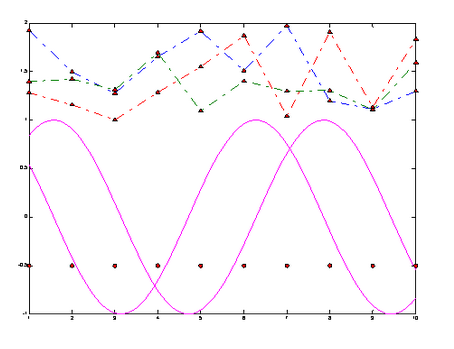举例如下：

定义x02pi之间的一组向量，且x向量中相邻两个值之间的增量为pi/100。定义y向量中的值为x向量的sine值。

x = 0:pi/100:2*pi;

y = sin(x);

figure 打开新的绘画窗口，可省略该句

plot(x,y) %采用默认样式，绘制实线

x = 0:pi/20:2*pi;

y = sin(x);

plot(x,y,'--or') %坐标点为圆圈标志，且线型为红色短划线的绘图样式

注：可以根据上述LineSpec表格自由组合线型样式。

在不关闭绘图窗口的前提下，还可以为图像添加标题，x轴和y轴标签，例如：

xlabel('x')

ylabel('sin(x)')

title('Plot of the Sine Function')

想要在现有的图片上添加另外一条线，可以使用hold语句，例如：

x = 0:pi/100:2*pi;

y = sin(x);

plot(x,y)

hold on

y2 = cos(x);

plot(x,y2,'r:')

legend('sin','cos') %为图片添加图例## 使用plot3绘制三维图像

MATLAB中有许多函数可以用来绘制三维图像，在此仅对plot3函数进行简要介绍，有兴趣的同学可以自行学习。plot3函数的调用形式如下：

plot3(X, Y, Z,LineSpec)

例如：

z=0:pi/50:10*pi;

x=sin(z);

y=cos(z);

plot3(x,y,z)

展开全文matlab
• matlab_plot函数用法 matlab_plot函数用法 matlab_plot函数用法 matlab_plot函数用法 matlab_plot函数用法 matlab_plot函数用法
• ## matlab中plot函数用法

万次阅读 多人点赞 2019-03-06 14:41:15
1.简单的2维直线图 : plot(x,y) 同一坐标显示n条线：plot(x,y1,x,y2，…) x = 0:pi/10:2*pi; y = sin(x); figure; hold on; plot(x,y) 2.plot(X):X是矩阵，表示矩阵的每一行都画一条线，将显示n条线。 X=rand(3,3)...matlab
• 1、 plot函数的基本用法 plot(x, y)：其中，x和y分别存储x坐标与y坐标。 代码示例： clc; clear all; x = [1,3,4,6]; %x数据 y = [2,3,4,5]; %y数据 plot(x,y); 运行结果： 2、最简单的plot函数的调用格式 plot(x...
• 三维绘图基本流程 三维绘图的基本流程如下： ...绘制二维折线或曲线时，可以使用plot命令。与这条命令类似，MATLAB也提供了一个绘制三维折线或曲线的基本命令plot3。 该命令的格式如下。 plot2(x1,y1,z1,optimatlab
• 文章目录0 前言1 plot3函数1.1 plot3函数的基本用法1.2 plot3（x，y，z）函数参数的变化形式1.3 含多组输入参数的plot3函数1.4 含选项的plot3函数2 fplot3函数2.1 fplot3函数的基本用法2.2 练习3 结语 0 前言 本文是...matlab
• ## Matlab的中Plot函数的基本用法(详解)

万次阅读 多人点赞 2019-05-14 15:23:02
本文接下来要讲解一下Matlab中最基本的Plot用法 Plot的定义和用法 此 MATLAB 函数 创建 Y 中数据对 X 中对应值的二维线图。 如果 X 和 Y 都是向量，则它们的长度必须相同。plot 函数绘制 Y 对 X 的图。 如果 X ...Matlab
• 文章目录前言一、plot()函数二、代码运行结果总结 前言 此为本人学习中的一些笔记总结，仅供参考。...3.plot(x,y1,x,y2,'--',x,y3,':') 表示绘制三条正弦曲线，第一条曲线使用默认的线型， 为第二
• (1)当x是实向量时，则绘制出以该向量元素的下标(即向量的长度，可用MATLAB函数length求得)为横坐标，以该向量元素的值为纵坐标的一条连续曲线。 >> x=[10,20,30,40,50]; >> plot(x) (2)当x是实矩阵时...matlab
• ## Python直接使用plot()函数画图

千次阅读 多人点赞 2022-04-05 20:41:57
一、plot（）函数的认识 在使用Python进行数据可视化编程中matplotlib库是我们用来对数据进行画图常用的第三方...初期学习直接使用plot（）函数能便于我们对后面图形学习奠定函数的参数及基础。 matplotlib图的组成：python
• 可以查看matplotlib的官网,里面有plot函数的各种用法,以及各种图像的实现方法,并且直接提供了实现的案例. [matplotlib官网(https://matplotlib.org/stable/api/_as_gen/matplotlib.pyplot.plot.html)python matplotlib plt
• 使用plot函数绘制图像 plot(x, y) 对图像属性进行设置，可以使用以下代码： p = plot(x, y); p.LineWidth = 2; %线宽为2 p.Color = 'r'; % 红色线条 p.Marker = '*' ; % 设置标记类型 p.MarkerSize = 6; %设置标记...matlab 开发语言 矩阵
• 在前文《Python直接使用plot()函数画图》提到了matplotlib库中最简单的一个函数plot()函数，介绍plot()最简单的使用方法，包括plot()函数中的参数，画布的基础设置，简单的数据代入画图，本次进阶使用，我们会将其中...python
•matplotlib
• Matlab中使用Plot函数动态画图方法.zip
• ## plot函数的用法

千次阅读 2014-09-03 15:45:10
功能 二维曲线绘图   ...plot(Y) ...plot(X1,Y1,...) ...plot(X1,Y1,LineSpec,...)...plot(...,'PropertyName',PropertyValue,...) plot(axes_handle,...) h = plot(...) hlines = plot('v6',...)   描述 pmatlab
• 使用plot 函数之前，必须首先定义好曲线上每一点的x 及y 坐标.下面简单介绍! 工具/原料 matlab2012b+win7.8 方法/步骤 1  plot(x) 当x 为一向量时，以x ...
• ## MATLAB：plot函数详解

万次阅读 多人点赞 2021-08-08 20:50:29
本文介绍了MATLAB中plot函数用法，方便日后适用
• 目的：将matlab二维数组通过plot3形象的三维图表达 成果图如上图所示，黑色的线，红色的线和绿色的线分别代表数据集中的三个标签类别，横轴代表样本总数90个（图中没有截全，...不熟悉plot3函数的朋友们可以先使用...matlab 二维数组
• Pandas 之所以能够实现了数据可视化，主要利用了 Matplotlib 库的 plot() 方法，它对 plot() 方法做了简单的封装。数据挖掘 python 大数据
• 这是如何从 MATLAB:registered: 中的函数创建线图的示例。 阅读 MATLAB 文档中的“fimplicit”函数。 此功能在 R2016b 或更新版本中可用。 有关更多示例，请转到 MATLAB 绘图库 - ...matlab...